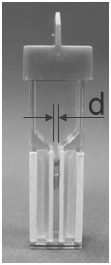Activate JavaScript to enable computations!

# Pulsed Power Toolbox

This is the start of a collection of Pulsed Power related formulas and calculators that I found usefull. However, whenever I could find resources on the internet already, I tried to refer to them rather than to re-invent the wheel. Consequently you might also find some of the links provided helpful.

## Parameters for Nanosecond Pulsed Electric Field Exposures

This calculator allows derivations for 'standard' experiments in electroporation cuvettes as they are common at the Center for Bioelectrics.
The calculator can not be used for more complex geometries and conditions such as used in in vitro experiments!

Standard
Electropoaration Cuvette

gap distance:
d = 1, 2, or 4 mmCalculation of Required Pulse Voltage:
 electric field wanted: E = kV/cm electrode separation: d = mm voltage needed: kV

Energy Delivered Per Pulse:
The calculation uses the input for electric field and gap distance above!
 pulse duration: τ = ns conductivity of buffer/suspension: σ = mS/cm energy: J
 energy density: J/cm3

Charge Transfered Per Pulse:
The calculation uses the input for electric field, gap distance, and conductivity above!
 charge: µAs

Temperature Increase of Suspension Per Pulse (adiabatic):
The calculation uses the input for electric field, gap distance, and conductivity above!
 temperature-change: K

Notes:

1. Calculation of the electric field for a given voltage and cuvette gap distance:
 applied voltage: V = kV gap distance: d = mm electric field: kV/cm
2. The pulse generators at the Frank Reidy Research Centers are usually hooked up to an oscilloscope with Tektronix voltage probe P6015A. Since we usually have not set a calibration value on the oscilloscope the 'real' voltage applied to the cuvette measured in kilovolts (kV) is observed on the oscilloscope in volts (V). This means, if you have/need for example a voltage of 10 kV applied to the cuvette, the pulse amplitud observed on the oscilloscope is 10 V.
3. The calculations here are only valid for the simple geometry of a cuvette filled with a liquid. More complex geometries, samples and experiments, such as used for example in in vitro experiments can not be analyzed with the calculators here.
4. The calculation of the temperature increase assumes a specific heat capacity of 4.2 kJ·kg-1·K-1, it is assumed that it is basically water that is heated. The heated volume is calculated automatically from the gap-distance and the known electrode area, which is the same for any electroporation cuvette independent from the manufacturer.
5. The transfered charge per pulse (used as scaling parameter for the charging of cell membranes "E·τ") is given in units of "µAs," i.e. "micro-amps-seconds," or equivalent "micro-coulombs."
6. Conversion from resistivities to conductivities:
 resistivity of suspension: ρ = Ω·cm conductivity: mS/cm cuvette resistance: Ω
For all practical purposes, can the conductivity of a cell-suspension be substituted by the conductivity of the buffer.
7. If any of the computations for energy, energy density, charge, or temperature change results in zero, then the calculated value is so small that it is negligible. (Smaller than two digits after the decimal point for the given unit.)